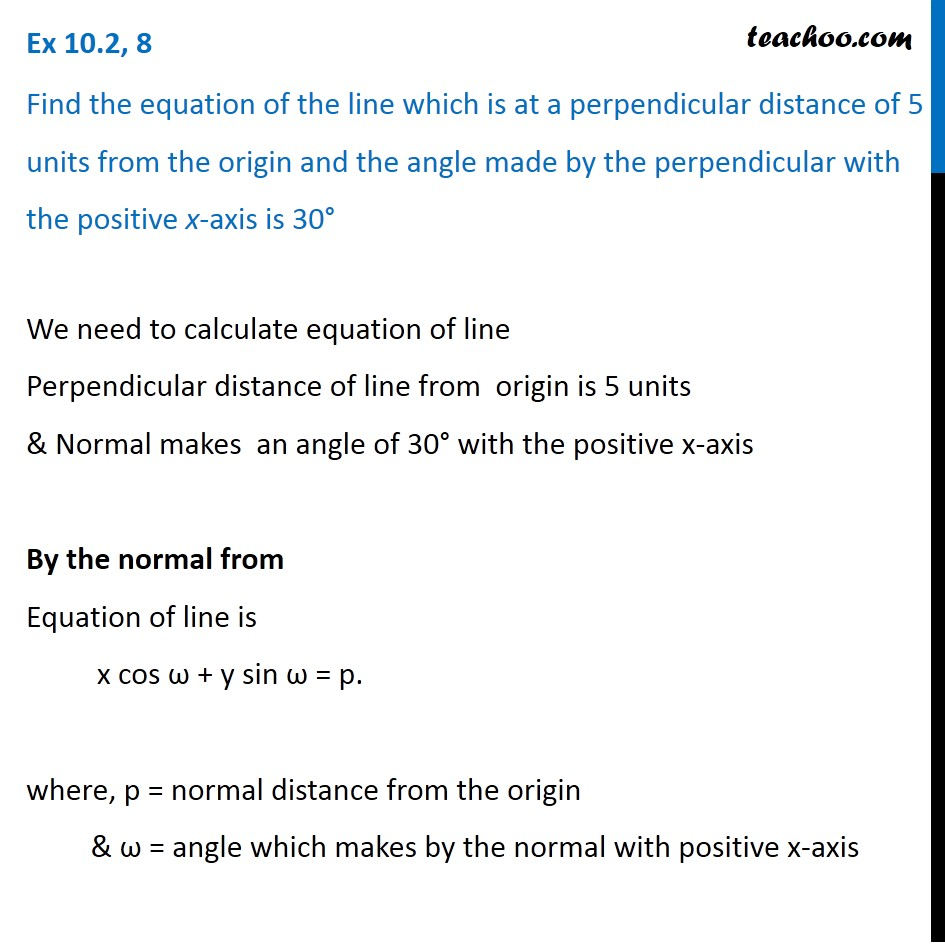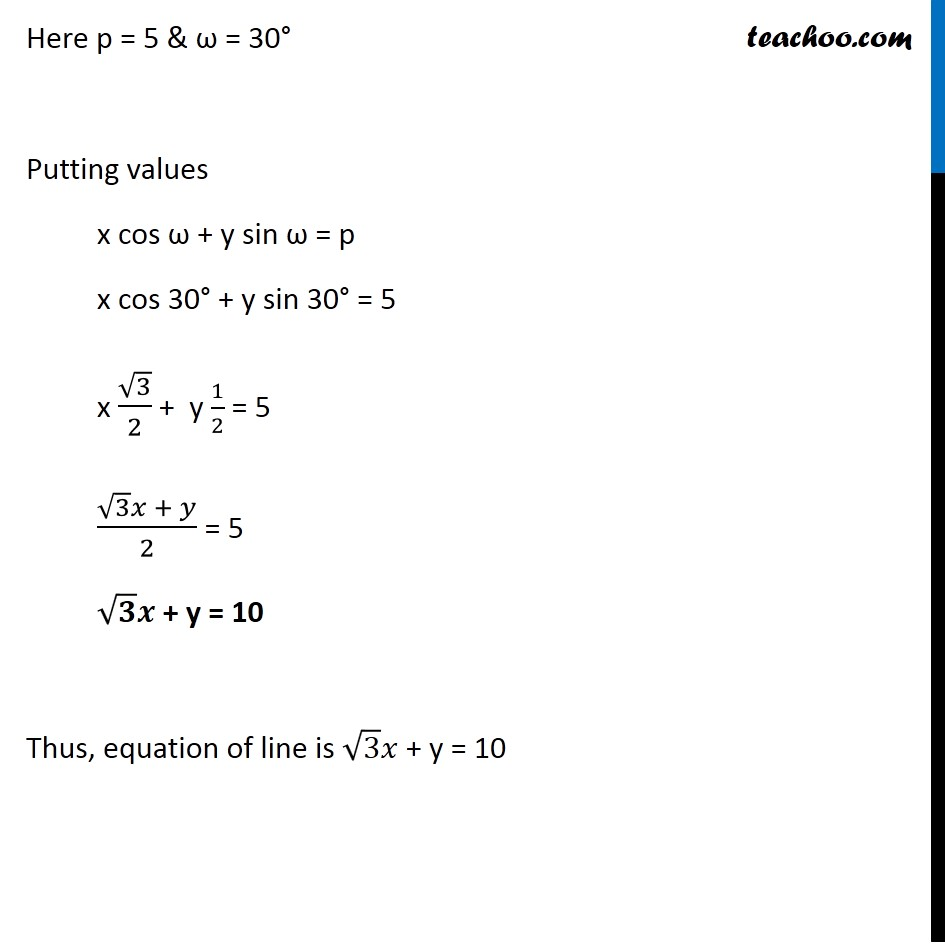Ex 9.2

Chapter 9 Class 11 Straight Lines
Serial order wiseLearn in your speed, with individual attention - Teachoo Maths 1-on-1 Class

### Transcript

Question 1 Find the equation of the line which is at a perpendicular distance of 5 units from the origin and the angle made by the perpendicular with the positive x-axis is 30° We need to calculate equation of line Perpendicular distance of line from origin is 5 units & Normal makes an angle of 30° with the positive x-axis By the normal from Equation of line is x cos ω + y sin ω = p. where, p = normal distance from the origin & ω = angle which makes by the normal with positive x-axis Here p = 5 & ω = 30° Putting values x cos ω + y sin ω = p x cos 30° + y sin 30° = 5 x √3/2 + y 1/2 = 5 (√3 𝑥 + 𝑦)/2 = 5 √𝟑 𝒙 + y = 10 Thus, equation of line is √3 𝑥 + y = 10